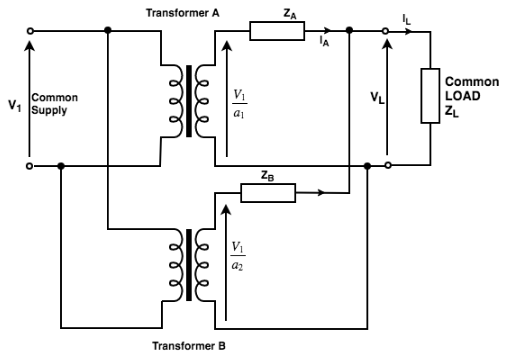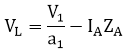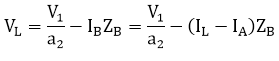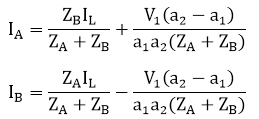Courses

# Parallel Operations of Transformers Electrical Engineering (EE) Notes | EduRev

## Electrical Engineering (EE) : Parallel Operations of Transformers Electrical Engineering (EE) Notes | EduRev

The document Parallel Operations of Transformers Electrical Engineering (EE) Notes | EduRev is a part of the Electrical Engineering (EE) Course Electrical Machines for Electrical Engg..
All you need of Electrical Engineering (EE) at this link: Electrical Engineering (EE)

When we connect the primary windings of two transformers to a common supply voltage and the secondary windings of both the transformers to a common load, this type of connection of transformer is said to be the parallel operation of transformers.

Reasons for parallel operation
The reasons for operating the transformers in parallel are as follows:

1. This is an economical method because a single large transformer is uneconomical for large load.
2. If the transformers are connected in parallel, we require extra load then we can expand the system by adding more transformers in the future.
3. Parallel operation reduces the space capacity of the substation when we connect transformers of standard size.
4. The parallel connection maximizes the electrical power system availability as we can shut down any system for maintenance without affecting other systems performance.

Single-phase transformers in parallel:
The diagram drawn below shows the circuit diagram of two transformers A and B connected in parallel.
Let,
a1 = turns ratio of transformer A
a2 = turns ratio of transformer B
ZA = equivalent impedance of transformer A referred to the secondary side.
ZB = equivalent impedance of transformer B referred to the secondary side.
ZL = load impedance across the secondary side.
IA = current supplied to the load by the secondary of transformer A.
IB = current supplied to the load by the secondary of transformer B.Fig: Two single-phase transformers in parallel.
By KCL,
IA + IB = IL
BY KVL,By solving the above two equations, we getEach of these currents has two components; the first component represents the transformer's share of the load current and the second component is a circulating current in the secondary windings.
Circulating currents have the following undesirable effects:

• They increase the copper loss.

Conditions for parallel operation of Single-Phase transformers:

Necessary conditions

1. The transformers must have the same polarities.
2. The transformers should have equal turn ratios.

Desirable conditions

• The voltages at full load across transformers internal impedance should be equal.
• The ratios of their winding resistances to reactances should be equal for both transformers. This condition ensures that both transformers operate at the same power factor, thus sharing active power and reactive voltamperes according to their ratings.

Three-phase transformers in parallel
The conditions for proper parallel operation of single-phase transformers are as follows:

• The polarities of the transformers should be the same.
• Identical primary and secondary voltage ratings.
• Impedances inversely proportional to the kVA ratings.
• Identical X/R ratios in the transformer impedances.

The condition for the parallel operation of single phase and three phase transformer is the same but with the following additions:

1. The phase sequence of the transformers must be identical.
2. The primary and secondary voltages of all the transformers connected in parallel must have the same phase shift.
Offer running on EduRev: Apply code STAYHOME200 to get INR 200 off on our premium plan EduRev Infinity!

## Electrical Machines for Electrical Engg.

22 videos|41 docs|26 tests

,

,

,

,

,

,

,

,

,

,

,

,

,

,

,

,

,

,

,

,

,

;